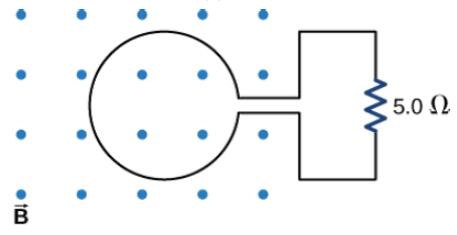# Problem: Lenz's Law The magnetic flux through the loop shown in the accompanying figure varies with time according to Φm = 2.00e-3tsin(120πt), where Φm is in milliwebers. What are the direction and magnitude of the current through the 5.00-Ω resistor at  t = 0s?

###### FREE Expert Solution

Induced emf:

$\overline{){\mathbf{\epsilon }}{\mathbf{=}}{\mathbf{-}}\frac{{\mathbf{d\varphi }}_{\mathbf{m}}}{\mathbf{dt}}}$

Current:

$\overline{){\mathbf{i}}{\mathbf{=}}\frac{\mathbf{\epsilon }\mathbf{\left(}\mathbf{t}\mathbf{\right)}}{\mathbf{R}}}$

According to Lenz's law, the value of the current is positive if it is in a counterclockwise direction.

98% (443 ratings)###### Problem Details

Lenz's Law

The magnetic flux through the loop shown in the accompanying figure varies with time according to Φm = 2.00e-3tsin(120πt), where Φm is in milliwebers. What are the direction and magnitude of the current through the 5.00-Ω resistor at  t = 0s?